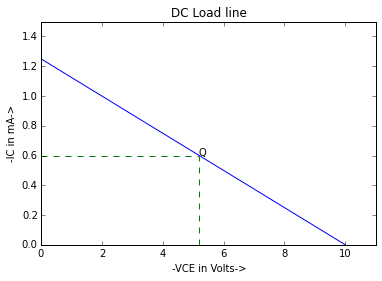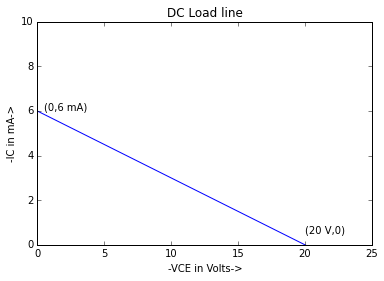# Chapter 6 , Bipolar Junction Trasistor¶

## Example 6.1 , Page Number 192¶

In :
#Variables

IE = 10 * 10**-3                 #Emitter current (in Ampere)
IC = 9.95 * 10**-3               #Collector current (in Ampere)

#Calculation

IB = IE - IC                     #Base current (in Ampere)

#Result

print "Base current : ",IB * 10**3,"mA."

Base current :  0.05 mA.


## Example 6.2 , Page Number 192¶

In :
#Variables

IB = 0.5 * 10**-3                 #Base current (in Ampere)
IC = 100.0 * 10**-3               #Collector current (in Ampere)

#Calculation

IE = IB + IC                      #Emitter current (in Ampere)
alphadc = IC/IE                   #Current amplification factor

#Result


Current gain (alphadc) :  0.995 .


## Example 6.3 , Page Number 193¶

In :
#Variables

IB = 50 * 10**-6                 #Base current (in Ampere)
ICBO = 4 * 10**-6                #Collector-to-base leakage current (in Ampere)
alphadc = 0.98                   #Current amplification factor

#Calculation

IE = IC + IB                               #Emitter current (in Ampere)

#Result

print "Emitter current : ",IE * 10**3," mA.\nCollector current : ",IC * 10**3," mA."

Emitter current :  2.7  mA.
Collector current :  2.65  mA.


## Example 6.4 , Page Number 193¶

In :
#Variables

IC = 20.0 * 10**-3                #Collector current (in Ampere)
beta = 50                         #Current gain

#Calculation

IB = IC/beta                      #Base current (in Ampere)
IE = IC + IB                      #Emitter current (in Ampere)

#Result

print "Emitter current : ",IE * 10**3,"mA."

Emitter current :  20.4 mA.


## Example 6.5 , Page Number 194¶

In :
#Variables

IB = 20.0 * 10**-6                 #Base current (in Ampere)
IC = 0.98 * 10**-3                 #Collector current (in Ampere)

#Calculation

IE = IB + IC                      #Emitter current (in Ampere)
alphadc = IC/IE                   #Current amplification factor
beta = IC/IB                      #Current gain

#Result

print "Emitter current : ",IE*10**3,"mA.\nCurrent Amplification factor : ",alphadc,".\nCurrent gain factor : ",beta,"."

Emitter current :  1.0 mA.
Current Amplification factor :  0.98 .
Current gain factor :  49.0 .


## Example 6.6 , Page Number 194¶

In :
#Variables

IB = 10 * 10**-6                   #Base current (in Ampere)
ICBO = 1.0 * 10**-6                #Collector-to-base leakage current (in Ampere)
beta = 99                          #Current amplification factor

#Calculation

IC = beta*IB + (1 + beta)*ICBO     #Collector current (in Ampere)

#Result

print "Collector current : ",IC*10**3," mA."

Collector current :  1.09  mA.


## Example 6.7 , Page Number 199¶

In :
#Variables

IC = -6.4               #Collector current (in milli-Ampere)
IE = 6.6                #Emitter current (in milli-Ampere)

#Calculation

alpha = -IC/IE          #Current amplification factor

#Result

print "Current amplification factor : ",round(alpha,4),"."

Current amplification factor :  0.9697 .


## Example 6.8 , Page Number 199¶

In :
#Variables

dVEB = 200 * 10**-3             #Change in emitter voltage
dIE  = 5 * 10**-3               #Change in emitter current

#Calculation

rin = dVEB/dIE                  #Dynamic input resistance (in ohm)

#Result

print "Dynamic input resistance : ",rin," ohm."

Dynamic input resistance :  40.0  ohm.


## Example 6.9 , Page Number 199¶

In :
#Variables

ICO = 10 * 10**-6           #Reverse saturation current (in Ampere)
IE = 2 * 10**-3             #Emitter current (in Ampere)
alpha = 0.98                #Current amplification factor

#Calculation

IC = alpha*IE + ICO         #Collector current (in Ampere)
IB = IE - IC                #Base current (in Ampere)

#Result

print "Base current : ",IB * 10**6," micro-A.\nCollector current : ",IC * 10**3," mA."

Base current :  30.0  micro-A.
Collector current :  1.97  mA.


## Example 6.10 , Page Number 199¶

In :
#Variables

IE = 2.0 * 10**-3                #Emitter current (in Ampere)
IC = 1.97 * 10**-3               #Collector current (in Ampere)
ICBO = 12.5 * 10**-6             #Reverse saturation current (in Ampere)

#Calculation

alpha = (IC-ICBO)/IE             #Current amplification factor
IB = IE - IC                     #Base current (in Ampere)

#Result

print "Current gain : ",round(alpha,3),".\nBase current : ",IB * 10**3,"mA."

Current gain :  0.979 .
Base current :  0.03 mA.


## Example 6.11 , Page Number 199¶

In :
#Variables

RL = 4.0 * 10**3               #Load resistance (in ohm)
VL = 3                         #Voltage drop across load (in volts)
alpha = 0.96                   #Current amplification factor

#Calculation

IC = VL/RL                     #Collector current (in Ampere)
IE = IC/alpha                  #Emitter current (in Ampere)
IB = IE - IC                   #Base current (in Ampere)

#Result

print "Base current : ",round(IB * 10**3,2),"mA."

Base current :  0.03 mA.


## Example 6.12 , Page Number 204¶

In :
#Variables

alpha1 = 0.99                          #Current gain1 in CB
beta2 = 80.0                           #Current gain2 in CE

#Calculation

beta1 = alpha1/(1-alpha1)              #Current gain1 in CE
alpha2 = beta2/(1 + beta2)             #Current gain2 in CB

#Result

print "Current gain in CE configuration : ",beta1,".\nCurrent gain in CB configuration : ",round(alpha2,3),"."

Current gain in CE configuration :  99.0 .
Current gain in CB configuration :  0.988 .


## Example 6.13 , Page Number 204¶

In :
#Variables

RL = 1.0 * 10**3                  #Load resistance (in ohm)
VL = 1.2                          #Voltage across load (in volts)
beta = 60                         #Current gain in CE

#Calculation

IC = VL/RL                        #Collector current (in Ampere)
IB = IC/beta                      #Base current (in Ampere)

#Result

print "Base current : ",IB * 10**6,"micro-A."

Base current :  20.0 micro-A.


## Example 6.14 , Page Number 204¶

In :
#Variables

VCC = 10.0                    #Collector supply voltage (in volts)
VL = 0.8                      #Voltage drop across load (in volts)
RL = 800                      #Load resistance (in ohm)
alpha = 0.96                  #Current gain in CB

#Calculation

VCE = VCC - VL                #Collector-emitter voltage (in volts)
IC = VL/RL                    #Collector current (in Ampere)
beta = alpha/(1-alpha)        #Current gain in CE
IB = IC/beta                  #Base current (in Ampere)

#Result

print "VCE : ",VCE,"V."
print "Base current : ",round(IB * 10**6,2)," micro-A."

VCE :  9.2 V.
Base current :  41.67  micro-A.


## Example 6.15 , Page Number 205¶

In :
#Variables

ICO = 10.0 * 10**-6              #Reverse saturation current (in Ampere)
alpha = 0.98                     #Current gain in CB
IB = 0.22 * 10**-3               #Base current (in Ampere)

#Calculation

IC = (alpha*IB + ICO)/(1-alpha)  #Collector current (in Ampere)

#Result

print "Collector current : ",IC * 10**3,"mA."

Collector current :  11.28 mA.


## Example 6.16 , Page Number 205¶

In :
#Variables

dVBE = 250 * 10**-3                 #Change in base-emitter voltage (in volts)
dIB = 1.0 * 10**-3                  #Change in base current (in Ampere)

#Calculation

rin = dVBE/dIB                      #Dynamic input resistance (in ohm)

#Result

print "Dynamic input resistance : ",rin," ohm."

Dynamic input resistance :  250.0  ohm.


## Example 6.17 , Page Number 205¶

In :
#Variables

dVCE = 5                            #Change in collector-emitter voltage (in volts)
dIC = 0.8 * 10**-3                  #Change in base current (in Ampere)

#Calculation

rout = dVCE/dIC                     #Dynamic output resistance (in ohm)

#Result

print "Dynamic output resistance : ",rout * 10**-3,"kilo-ohm."

Dynamic output resistance :  6.25 kilo-ohm.


## Example 6.18 , Page Number 209¶

In :
import math
import numpy
%matplotlib inline
from matplotlib.pyplot import plot,title,xlabel,ylabel,ylim,xlim,annotate

#Variables

VCC = 10.0                              #Collector supply voltage (in volts)
RC = 8.0 * 10**3                        #Load resistance (in ohm)
IB = 15.0 * 10**-6                      #Base current (in Ampere)
beta = 40                               #Current gain in CE

#Calculation

IC = VCC/RC                             #Collector current (in Ampere)
IC1 = beta * IB                         #Zero signal collector current (in Ampere)
VCE = VCC - IC1*RC                      #Zero signal collector-emitter voltage (in volts)

#Result

print "Operating point Q is (",VCE,"V ,",IC1 * 10**3,"mA.)"

#Graph

x = numpy.linspace(0,10,100)
y1 = numpy.linspace(0,0.6,100)
x1 = numpy.linspace(0,5.2,100)
plot(x,1.25-1.25/10*x,'b')
plot(x1,0.6+x1-x1,'--',color='g')
plot(5.2+y1-y1,y1,'--',color='g')
annotate('Q',xy=(5.2,0.6))
xlim(0,11)
ylim(0,1.5)
xlabel("-VCE in Volts->")
ylabel("-IC in mA->")

Operating point Q is ( 5.2 V , 0.6 mA.)

Out:
<matplotlib.text.Text at 0x642f590>## Example 6.19 , Page Number 210¶

In :
#Variables

IC = 1.2 * 10**-3             #Collector current (in Ampere)
RL = 5.0 * 10**3              #Load resistance (in ohm)
VCC = 12.0                    #Collector supply voltage (in volts)

#Calculation

VCE = VCC - IC*RL             #Zero signal collector-emitter voltage (in volts)
RL1 = 7.5 * 10**3             #Changed load resistance (in ohm)
VCE1 = VCC - IC*RL1           #Changed zero signal collector-emitter voltage (in volts)

#Result

print "Operating point  is (",VCE,"V,",IC*10**3,"mA )."
print "Changed operating point is (",VCE1,"V,",IC*10**3,"mA )."

Operating point  is ( 6.0 V, 1.2 mA ).
Changed operating point is ( 3.0 V, 1.2 mA ).


## Example 6.20 , Page Number 210¶

In :
import math
import numpy
%matplotlib inline
from matplotlib.pyplot import plot,title,xlabel,ylabel,ylim,xlim

#Variables

IC = 0                       #Collector current (in Ampere)
VCE = VCC = 20               #Collector supply (in volts)
RC = 3.3 * 10**3             #Resistance in collector branch (in ohm)

#Calculation

VCE1 = 0                     #Saturation point collector-emitter voltage (in volts)
IC = VCC/RC                  #Collector current at saturation point (in Ampere)

#Result

print "At cut-off point VCE :",VCE,"V."
print "At saturation point IC :",round(IC * 10**3),"mA."

#Graph

x = numpy.linspace(0,25,100)
plot(x,6-6.0/20*x,'b')
annotate('(0,6 mA)',xy=(0.5,6))
annotate('(20 V,0)',xy=(20,0.5))
xlim(0,25)
ylim(0,10)
xlabel("-VCE in Volts->")
ylabel("-IC in mA->")

At cut-off point VCE : 20 V.
At saturation point IC : 6.0 mA.

Out:
<matplotlib.text.Text at 0x721b590>## Example 6.21 , Page Number 210¶

In :
#Variables

VCC = 0                         #Collector supply (in volts)
beta = 45.0                     #Current gain in CE
VBE = 0.7                       #Emitter-base voltage (in volts)
VEE = 9                         #Emitter supply (in volts)
RB = 100 * 10**3                #Resistance in base branch (in ohm)
RC = 1.2 * 10**3                #Resistance in collector branch (in ohm)

#Calculation

IB = (VEE - VBE)/RB             #Base current (in Ampere)
IC = beta * IB                  #Collector current (in Ampere)
VC = VCC - IC * RC              #Collector voltage (in volts)
VB = VBE + VEE                  #Base voltage (in volts)

#Result

print "VC for the network :",VC,"V."
print "VB for the network :",VB,"V."

#Slight variation due to higher precision.

VC for the network : -4.482 V.
VB for the network : 9.7 V.


## Example 6.22 , Page Number 211¶

In :
#Variables

VCC = 10                        #Collector supply (in volts)
VBE = 0.8                       #Emitter-to-base voltage (in volts)
VCE = 0.2                       #Collector-to-emitter voltage (in volts)
beta = hfe = 100                #Current gain in CE
VBB = 5                         #base supply (in volts)
RB = 50 * 10**3                 #Resistance in base branch (in ohm)
RE = 2 * 10**3                  #Resistance in emitter branch (in ohm)
RC = 3 * 10**3                  #Resistance in collector branch (in ohm)

#Calculation

IB = (VBB - VBE)/(RB+(1+beta)*RE)   #Base current (in Ampere)
IC = (VCC - VCE)/(RE + RC)          #Collector current (in Ampere)

#Result

print "IB : ",round(IB* 10**3,4),"mA."
print "IC : ",IC* 10**3,"mA."
print "Since, beta * IB < IC , therefore , transistor is in saturation."

IB :  0.0167 mA.
IC :  1.96 mA.
Since, beta * IB < IC , therefore , transistor is in saturation.


## Example 6.23 , Page Number 215¶

In :
#Variables

Tf = 105                   #Free air temp. (in Celsius degree)
Tf1 = 80                   #Temp. in excess of 25 degree celsius (in Celsius degree)
df = 2.81                  #derating factor  (in milli-Watt per Celsius degree)
VCE = 20                   #Collector-to-emitter voltage (in volts)
Porig = 310.0              #Original maximum power dissipation (in milli-Watt)

#Calculation

Pcmax  = Porig - Tf1 * df  #Derated power dissipation (in milli-watt)
ICmax = Pcmax/VCE          #Device dissipation (in Ampere)

#Result

print "Maximum level of collector current : ",ICmax," mA."

#Calculation error in book.

Maximum level of collector current :  4.26  mA.